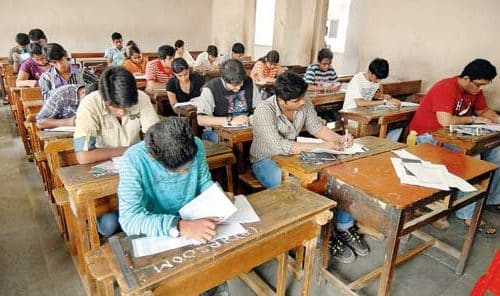### Part C: Quantitative Aptitude

• Questions will be designed to test the ability of appropriate use of numbers and number sense of the candidate.
• The scope of the test will be:
1. Computation of whole numbers, decimals, fractions and relationship between numbers
2. Percentage
3. Ratio and Proportion
4. Square Roots
5. Averages
6. Interest
7. Profit % Loss
8. Discount
9. Partnership Business
10. Mixture & Allegation
11. Time & Distance
12. Time & Work
13. Basic Algebraic identities of School Algebra & Elementary Surds
14. Graphs of Linear Equations
15. Triangle and its various kind of centres, congruence & similarity of triangles
16. Circle & its chords, tangents, angles subtended by chords of a circle, common tangents to two or more circles.
17. Triangle
18. Quadrilaterals
19. Regular Polygons
20. Circle
21. Right Prism
22. Right Circular Cone
23. Right Circular Cylinder
24. Sphere, Hemisphere
25. Rectangular Parallelepiped
26. Regular Right Pyramid with triangular or square base
27. Trigonometric Ratio
28. Degree and Radian Measures
29. Standard Identities
30. Complementary Angles
31. Heights & Distances
32. Histogram
33. Frequency Polygon
34. Bar diagram
35. Pie Chart

### Part D: English Comprehension

• This section will test candidate’s ability to understand English language.